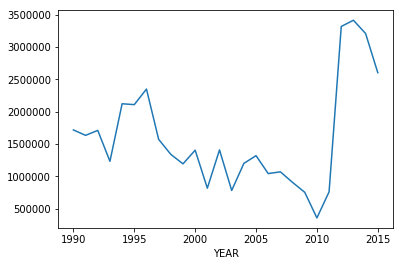## Exploratory analysis of the US Airport Dataset¶

This dataset contains data for 25 years[1995-2015] of flights between various US airports and metadata about these routes. Taken from Bureau of Transportation Statistics, United States Department of Transportation.

Let's see what can we make out of this!

In :
%matplotlib inline
import networkx as nx
import pandas as pd
import matplotlib.pyplot as plt
import numpy as np
import warnings
warnings.filterwarnings('ignore')



In the pass_air_data dataframe we have the information of number of people that fly every year on a particular route on the list of airlines that fly that route.

In :
pass_air_data.head()

Out:
Unnamed: 0 YEAR ORIGIN DEST UNIQUE_CARRIER_NAME PASSENGERS
0 0 1990 ABE ACY {'US Airways Inc.'} 73.0
1 1 1990 ABE ATL {'Eastern Air Lines Inc.'} 73172.0
2 2 1990 ABE AVL {'Westair Airlines Inc.'} 0.0
3 3 1990 ABE AVP {'Westair Airlines Inc.', 'US Airways Inc.', '... 8397.0
4 4 1990 ABE BHM {'Eastern Air Lines Inc.'} 59.0
In :
# Create a MultiDiGraph from this dataset

passenger_graph = nx.from_pandas_edgelist(pass_air_data, source='ORIGIN', target='DEST', edge_attr=['YEAR', 'PASSENGERS', 'UNIQUE_CARRIER_NAME'], create_using=nx.MultiDiGraph())


### Cleveland to Chicago, how many people fly this route?¶

In :
passenger_graph['CLE']['ORD']

Out:
{'PASSENGERS': 409943.0,
'UNIQUE_CARRIER_NAME': "{'Frontier Airlines Inc.', 'SkyWest Airlines Inc.', 'Republic Airlines', 'ExpressJet Airlines Inc.', 'American Airlines Inc.', 'Swift Air, LLC', 'GoJet Airlines LLC d/b/a United Express', 'Trans States Airlines', 'Envoy Air', 'Spirit Air Lines', 'United Air Lines Inc.', 'Shuttle America Corp.'}",
'YEAR': 2015}
In :
temp = [(i['YEAR'], i['PASSENGERS'])for i in dict(passenger_graph['CLE']['ORD']).values()]
x, y = zip(*temp)
plt.plot(x, y)
plt.show()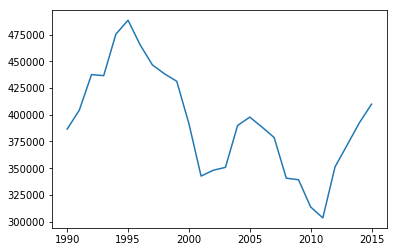## Exercise¶

Find the busiest route in 1990 and in 2015 according to number of passengers, and plot the time series of number of passengers on these routes.

You can use the DataFrame instead of working with the network. It will be faster ;) [5 mins]

In :
temp = pass_air_data.groupby(['YEAR'])['PASSENGERS'].transform(max) == pass_air_data['PASSENGERS']

In :
pass_air_data[temp][pass_air_data.YEAR.isin([1990, 2015])]

Out:
Unnamed: 0 YEAR ORIGIN DEST UNIQUE_CARRIER_NAME PASSENGERS
3917 3917 1990 LAX HNL {'Heavylift Cargo Airlines Lt', 'Hawaiian Airl... 1827164.0
445978 445978 2015 LAX SFO {'Hawaiian Airlines Inc.', 'Delta Air Lines In... 1869066.0
In :
pass_air_data[(pass_air_data['ORIGIN'] == 'LAX') & (pass_air_data['DEST'] == 'HNL')].plot('YEAR', 'PASSENGERS')

Out:
<matplotlib.axes._subplots.AxesSubplot at 0x1515268be0>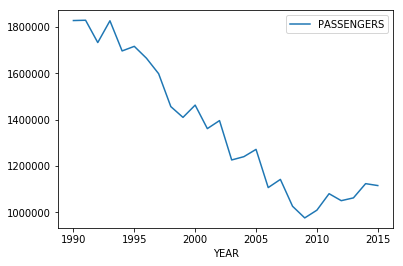In :
pass_air_data[(pass_air_data['ORIGIN'] == 'LAX') & (pass_air_data['DEST'] == 'SFO')].plot('YEAR', 'PASSENGERS')

Out:
<matplotlib.axes._subplots.AxesSubplot at 0x15224e7ef0>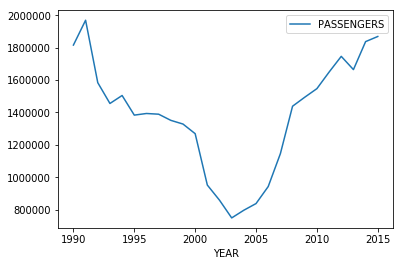So let's have a look at the important nodes in this network, i.e. important airports in this network. We'll use pagerank, betweenness centrality and degree centrality.

In :
# nx.pagerank(passenger_graph)

In :
def year_network(G, year):
temp_g = nx.DiGraph()
for i in G.edges(data=True):
if i['YEAR'] == year:
return temp_g

In :
pass_2015 = year_network(passenger_graph, 2015)

In :
len(pass_2015)

Out:
1258
In :
len(pass_2015.edges())

Out:
25354
In :
# Load in the GPS coordinates of all the airports

In :
lat_long[lat_long.isin(list(pass_2015.nodes()))]

Out:
0 1 2 3 4 5 6 7 8 9 10 11 12 13 14 15
1321 EGYC CLF COLTISHALL COLTISHALL ENGLAND 52 45 17 N 1 21 26 E 21 52.755 1.357
3380 KABI ABI ABILENE RGNL ABILENE USA 32 24 40 N 99 40 54 W 546 32.411 -99.682
3381 KABQ ABQ NaN ALBUQUERQUE USA 0 0 0 U 0 0 0 U 0 0.000 0.000
3382 KACK ACK NANTUCKET MEM NANTUCKET USA 41 15 10 N 70 3 36 W 15 41.253 -70.060
3383 KACT ACT WACO RGNL WACO USA 31 36 40 N 97 13 49 W 158 31.611 -97.230
3384 KACY ACY ATLANTIC CITY INTERNATIONAL ATLANTIC CITY USA 39 27 27 N 74 34 37 W 23 39.458 -74.577
3386 KADW ADW ANDREWS AFB CAMP SPRINGS USA 38 48 38 N 76 52 1 W 86 38.811 -76.867
3387 KAEX AEX ALEXANDRIA INTERNATIONAL ALEXANDRIA USA 31 19 38 N 92 32 54 W 28 31.327 -92.548
3389 KAGS AGS AUGUSTA RGNL AT BUSH FLD BUSH FIELD USA 33 22 11 N 81 57 52 W 44 33.370 -81.964
3391 KALB ALB ALBANY INTERNATIONAL ALBANY USA 42 44 53 N 73 48 10 W 87 42.748 -73.803
3394 KAMA AMA AMARILLO INTERNATIONAL AMARILLO USA 35 13 9 N 101 42 21 W 1100 35.219 -101.706
3395 KANB ANB ANNISTON METROPOLITAN ANNISTON USA 33 35 17 N 85 51 29 W 187 33.588 -85.858
3397 KAOO AOO ALTOONA BLAIR CO ALTOONA USA 40 17 47 N 78 19 12 W 459 40.296 -78.320
3399 KARA ARA ACADIANA REGIONAL LOUISIANA USA 30 2 15 N 91 53 2 W 8 30.038 -91.884
3400 KART ART WATERTOWN INTERNATIONAL WATERTOWN USA 43 59 30 N 76 1 18 W 100 43.992 -76.022
3401 KATL ATL THE WILLIAM B HARTSFIELD ATLANTA INTERNATIONAL ATLANTA USA 33 38 25 N 84 25 37 W 313 33.640 -84.427
3402 KAUG AUG AUGUSTA STATE AUGUSTA USA 44 19 14 N 69 47 50 W 108 44.321 -69.797
3403 KAUS AUS AUSTIN BERGSTROM INTERNATIONAL AUSTIN USA 30 11 40 N 97 40 11 W 166 30.194 -97.670
3406 KBAD BAD BARKSDALE AFB SHREVEPORT USA 32 30 6 N 93 39 45 W 51 32.502 -93.663
3407 KBCT BCT BOCA RATON BOCA RATON USA 26 22 42 N 80 6 27 W 4 26.378 -80.107
3409 KBDL BDL BRADLEY INTERNATIONAL WINDSOR LOCKS USA 41 56 20 N 72 40 59 W 53 41.939 -72.683
3410 KBDR BDR IGOR I SIKORSKY MEM STRATFORD USA 41 9 48 N 73 7 34 W 3 41.163 -73.126
3411 KBED BED LAURENCE G HANSCOM FLD BEDFORD USA 42 28 11 N 71 17 20 W 41 42.470 -71.289
3412 KBFI BFI BOEING FLD KING CO INTERNATIONAL SEATTLE USA 47 31 47 N 122 18 7 W 6 47.530 -122.302
3413 KBFL BFL MEADOWS FLD BAKERSFIELD USA 35 26 0 N 119 3 24 W 155 35.433 -119.057
3414 KBFM BFM MOBILE DOWNTOWN MOBILE USA 30 37 35 N 88 4 4 W 8 30.626 -88.068
3415 KBGR BGR BANGOR INTERNATIONAL BANGOR USA 44 48 26 N 68 49 41 W 59 44.807 -68.828
3417 KBHM BHM BIRMINGHAM INTERNATIONAL BIRMINGHAM USA 33 33 46 N 86 45 12 W 197 33.563 -86.753
3418 KBIF BIF BIGGS AAF EL PASO USA 31 50 58 N 106 22 48 W 1203 31.849 -106.380
3421 KBLI BLI BELLINGHAM INTERNATIONAL BELLINGHAM USA 48 47 33 N 122 32 15 W 52 48.792 -122.537
... ... ... ... ... ... ... ... ... ... ... ... ... ... ... ... ...
6624 PAUN UNK UNALAKLEET UNALAKLEET USA 63 53 18 N 160 47 56 W 7 63.888 -160.799
6625 PAVD VDZ VALDEZ PIONEER FIELD VALDEZ USA 61 8 2 N 146 14 54 W 37 61.134 -146.248
6627 PAWT AIN WAINWRIGHT AS FORT WAINWRIGHT USA 70 36 48 N 159 51 37 W 11 70.613 -159.860
6629 PAYA YAK YAKUTAT YAKUTAT USA 59 30 11 N 139 39 36 W 12 59.503 -139.660
6633 PFYU FYU FORT YUKON FORT YUKON USA 66 34 17 N 145 15 1 W 132 66.571 -145.250
6638 PGRO ROP ROTA INTERNATIONAL ROTA MARIANA ISLANDS 14 10 28 N 145 14 36 E 186 14.174 145.243
6639 PGSN SPN SAIPAN INTERNATIONAL SAIPAN MARIANA ISLANDS 15 7 10 N 145 43 45 E 66 15.119 145.729
6641 PGUA UAM ANDERSEN AFB ANDERSEN MARIANA ISLANDS 13 35 2 N 144 55 48 E 192 13.584 144.930
6642 PGUM GUM GUAM INTERNATIONAL AGANA MARIANA ISLANDS 13 29 2 N 144 47 49 E 91 13.484 144.797
6649 PHHN HNM HANA HANA USA 20 47 44 N 156 0 51 W 24 20.796 -156.014
6652 PHJH JHM KAPALUA LAHANIA-KAPALUA USA 20 57 46 N 156 40 27 W 79 20.963 -156.674
6655 PHKO KOA KONA INTERNATIONAL AT KEAHOLE KONA USA 19 44 19 N 156 2 44 W 15 19.739 -156.046
6658 PHLI LIH LIHUE LIHUE USA 21 58 34 N 159 20 19 W 47 21.976 -159.339
6659 PHMK MKK MOLOKAI MOLOKAI USA 21 9 10 N 157 5 46 W 139 21.153 -157.096
6661 PHMU MUE WAIMEA KOHALA KAMUELA USA 20 0 4 N 155 40 5 W 815 20.001 -155.668
6665 PHNG NGF KANEOHE BAY MCAF KANEOHE BAY USA 21 26 57 N 157 46 4 W 6 21.449 -157.768
6666 PHNL HNL HONOLULU INTERNATIONAL HONOLULU USA 21 18 57 N 157 55 36 W 4 21.316 -157.927
6667 PHNY LNY LANAI LANAI USA 20 47 8 N 156 57 5 W 399 20.786 -156.951
6668 PHOG OGG KAHULUI KAHULUI USA 20 53 55 N 156 25 50 W 17 20.899 -156.431
6670 PHTO ITO HILO INTERNATIONAL HILO USA 19 43 13 N 155 2 55 W 12 19.720 -155.049
6686 PPIZ PIZ POINT LAY LRRS POINT LAY USA 69 43 58 N 163 0 19 W 8 69.733 -163.005
8020 TIST STT CYRIL E KING ST. THOMAS VIRGIN ISL. 18 20 14 N 64 58 24 W 8 18.337 -64.973
8021 TISX STX HENRY E ROHLSEN ST. CRIOX ISLAND VIRGIN ISL. 17 42 6 N 64 47 54 W 20 17.702 -64.798
8022 TJBQ BQN RAFAEL HERNANDEZ AGUADILLA PUERTO RICO 18 29 41 N 67 7 46 W 73 18.495 -67.129
8023 TJCG VQS NaN VIEQUES PUERTO RICO 0 0 0 U 0 0 0 U 0 0.000 0.000
8024 TJCP CPX NaN CULEBRA PUERTO RICO 0 0 0 U 0 0 0 U 0 0.000 0.000
8027 TJIG SIG FERNANDO LUIS RIBAS DOMINICCI SAN JUAN PUERTO RICO 18 27 24 N 66 5 53 W 4 18.457 -66.098
8028 TJMZ MAZ EUGENIO MARIA DE HOSTOS MAYAGUEZ PUERTO RICO 18 15 20 N 67 8 54 W 9 18.256 -67.148
8030 TJPS PSE MERCEDITA PONCE PUERTO RICO 18 0 29 N 66 33 46 W 8 18.008 -66.563
8031 TJSJ SJU LUIS MUNOZ MARIN INTERNATIONAL SAN JUAN PUERTO RICO 18 26 21 N 66 0 6 W 3 18.439 -66.002

371 rows × 16 columns

In :
pos_dict = {}
for airport in lat_long[lat_long.isin(list(pass_2015.nodes()))].iterrows():
pos_dict[airport] = (airport, airport)

In :
pos_dict

Out:
{'ABI': (-99.682, 32.411),
'ABQ': (-106.609, 35.04),
'ABY': (0.0, 0.0),
'ACK': (-70.06, 41.253),
'ACT': (-97.23, 31.611),
'ACY': (-74.577, 39.458),
'AEX': (-92.54799999999999, 31.326999999999998),
'AGS': (-81.964, 33.37),
'AIN': (-159.86, 70.613),
'AKN': (-156.649, 58.677),
'ALB': (-73.803, 42.748000000000005),
'AMA': (-101.706, 35.219),
'ANB': (-85.85799999999999, 33.588),
'ANC': (-149.996, 61.174),
'AOO': (-78.32, 40.296),
'ARA': (-91.884, 30.038),
'ART': (-76.02199999999999, 43.992),
'ATL': (-84.427, 33.64),
'AUG': (-69.797, 44.321000000000005),
'AUS': (-97.67, 30.194000000000003),
'BCT': (-80.107, 26.378),
'BDL': (-72.683, 41.93899999999999),
'BDR': (-73.126, 41.163000000000004),
'BED': (-71.289, 42.47),
'BET': (-161.838, 60.78),
'BFI': (-122.302, 47.53),
'BFL': (-119.057, 35.433),
'BFM': (-88.068, 30.625999999999998),
'BGR': (-68.828, 44.806999999999995),
'BHM': (-86.75299999999999, 33.563),
'BIF': (-106.38, 31.849),
'BLI': (-122.537, 48.792),
'BLV': (-89.835, 38.545),
'BNA': (-86.678, 36.124),
'BOI': (-116.223, 43.56399999999999),
'BOS': (-71.005, 42.364),
'BPT': (-94.021, 29.951),
'BQK': (0.0, 0.0),
'BQN': (-67.12899999999999, 18.495),
'BRO': (-97.426, 25.906999999999996),
'BRW': (-156.766, 71.285),
'BTI': (-143.577, 70.134),
'BTR': (-91.149, 30.533),
'BTT': (-151.528, 66.915),
'BTV': (-73.153, 44.472),
'BUF': (-78.732, 42.94),
'BUR': (-118.359, 34.201),
'BWI': (-76.668, 39.175),
'CAE': (-81.119, 33.939),
'CDB': (-162.724, 55.206),
'CDC': (-113.09899999999999, 37.701),
'CDV': (-145.477, 60.492),
'CEF': (-72.53399999999999, 42.198),
'CEW': (-86.522, 30.779),
'CHA': (-85.204, 35.035),
'CHS': (-80.04, 32.899),
'CIC': (-121.85799999999999, 39.795),
'CLE': (-81.85, 41.412),
'CLF': (1.357, 52.755),
'CLL': (-96.36399999999999, 30.588),
'CLT': (-80.943, 35.214),
'CMH': (-82.89200000000001, 39.998000000000005),
'CNM': (-104.26299999999999, 32.336999999999996),
'COS': (-104.7, 38.806),
'COU': (-92.219, 38.818000000000005),
'CPR': (-106.464, 42.908),
'CPX': (0.0, 0.0),
'CRP': (-97.501, 27.77),
'CVG': (-84.662, 39.046),
'CVS': (-103.322, 34.383),
'CYS': (-104.81200000000001, 41.156000000000006),
'DAL': (-96.852, 32.847),
'DAY': (-84.219, 39.902),
'DCA': (-77.03699999999999, 38.852),
'DEN': (-104.667, 39.858000000000004),
'DET': (-83.01, 42.409),
'DFW': (-97.037, 32.896),
'DHN': (-85.449, 31.320999999999998),
'DLG': (-158.503, 59.045),
'DLH': (-92.194, 46.842),
'DMA': (-110.883, 32.166),
'DOV': (-75.46600000000001, 39.13),
'DPA': (-88.249, 41.908),
'DRO': (-107.75399999999999, 37.150999999999996),
'DRT': (-100.926, 29.373),
'DSM': (-93.663, 41.534),
'DTW': (-83.353, 42.211999999999996),
'DUT': (-166.543, 53.9),
'EDF': (-149.806, 61.251000000000005),
'EDW': (-117.884, 34.905),
'EFD': (-95.15899999999999, 29.607),
'EHM': (-162.061, 58.647),
'EIL': (-147.101, 64.666),
'ELD': (-92.81299999999999, 33.221),
'ELP': (-106.37799999999999, 31.807),
'ENA': (-151.245, 60.573),
'ENV': (-114.031, 40.719),
'EWN': (-77.043, 35.073),
'EWR': (-74.169, 40.692),
'EYW': (-81.759, 24.555999999999997),
'FAI': (-147.856, 64.815),
'FAT': (-119.71799999999999, 36.775999999999996),
'FBK': (-147.614, 64.837),
'FFO': (-84.04799999999999, 39.826),
'FLL': (-80.153, 26.072),
'FLO': (-79.72399999999999, 34.185),
'FMH': (-70.521, 41.658),
'FMN': (-108.23, 36.741),
'FMY': (-81.863, 26.586),
'FOD': (-94.193, 42.551),
'FOE': (-95.664, 38.951),
'FOK': (-72.632, 40.844),
'FSI': (-98.402, 34.65),
'FSM': (-94.367, 35.336),
'FTW': (-97.36200000000001, 32.82),
'FXE': (-80.171, 26.197),
'FYU': (-145.25, 66.571),
'FYV': (-94.17, 36.005),
'GAL': (-156.937, 64.736),
'GCK': (-100.72399999999999, 37.927),
'GEG': (-117.53399999999999, 47.62),
'GFK': (-97.176, 47.949),
'GGG': (-94.711, 32.385),
'GKN': (-145.45600000000002, 62.155),
'GNV': (-82.272, 29.69),
'GRB': (-88.12899999999999, 44.485),
'GRF': (-122.581, 47.07899999999999),
'GRK': (-97.829, 31.066999999999997),
'GRR': (-85.523, 42.881),
'GSB': (-77.961, 35.339),
'GTF': (-111.37100000000001, 47.482),
'GUM': (144.797, 13.484000000000002),
'GUS': (-86.152, 40.648),
'GVT': (-96.065, 33.068000000000005),
'HFD': (-72.65, 41.736000000000004),
'HIB': (-92.839, 47.386),
'HKY': (-81.389, 35.741),
'HLN': (-111.98299999999999, 46.607),
'HMN': (-106.10600000000001, 32.852),
'HNL': (-157.92700000000002, 21.316),
'HNM': (-156.014, 20.796),
'HOB': (-103.21700000000001, 32.688),
'HOM': (-151.476, 59.646),
'HON': (-98.228, 44.385),
'HOP': (-87.49600000000001, 36.668),
'HOU': (-95.279, 29.645),
'HPN': (-73.707, 41.067),
'HRL': (-97.654, 26.228),
'HRO': (-93.155, 36.260999999999996),
'HUF': (-87.307, 39.451),
'HVR': (-109.762, 48.543),
'IAB': (-97.26700000000001, 37.623000000000005),
'IAG': (-78.946, 43.107),
'IAH': (-95.34, 29.98),
'ICT': (-97.43299999999999, 37.65),
'ILG': (-75.60600000000001, 39.679),
'ILI': (-154.911, 59.754),
'ILM': (-77.903, 34.271),
'IND': (-86.294, 39.717),
'INL': (-93.40299999999999, 48.566),
'INT': (-80.222, 36.134),
'IPL': (-115.579, 32.834),
'IPT': (-76.921, 41.242),
'ISN': (-103.64200000000001, 48.178000000000004),
'ISP': (-73.1, 40.795),
'ITO': (-155.049, 19.72),
'JAN': (-90.07600000000001, 32.311),
'JAX': (-81.688, 30.494),
'JBR': (-90.646, 35.832),
'JFK': (-73.779, 40.64),
'JHM': (-156.674, 20.963),
'JNU': (-134.576, 58.355),
'KOA': (-156.046, 19.739),
'KTN': (-131.714, 55.356),
'LAN': (-84.587, 42.778999999999996),
'LAS': (-115.152, 36.08),
'LAX': (-118.40799999999999, 33.942),
'LBB': (-101.823, 33.664),
'LCH': (-93.223, 30.125999999999998),
'LCK': (-82.928, 39.814),
'LFI': (-76.36, 37.083),
'LFT': (-91.98700000000001, 30.205),
'LGA': (-73.872, 40.777),
'LGB': (-118.15100000000001, 33.818000000000005),
'LIH': (-159.339, 21.976),
'LIT': (-92.22399999999999, 34.729),
'LNK': (-96.759, 40.851),
'LNY': (-156.951, 20.785999999999998),
'LRD': (-99.461, 27.544),
'LSF': (-84.991, 32.336999999999996),
'LSV': (-115.03399999999999, 36.236),
'LUK': (-84.419, 39.103),
'LUR': (-166.11, 68.875),
'MAF': (-102.20200000000001, 31.941999999999997),
'MAZ': (-67.148, 18.256),
'MCC': (-121.40100000000001, 38.667),
'MCF': (-82.521, 27.849),
'MCG': (-155.606, 62.953),
'MCI': (-94.714, 39.297),
'MCN': (-83.649, 32.693000000000005),
'MCO': (-81.316, 28.429000000000002),
'MDT': (-76.763, 40.193000000000005),
'MDW': (-87.75200000000001, 41.786),
'MEM': (-89.977, 35.042),
'MER': (-120.568, 37.38),
'MFE': (-98.23899999999999, 26.176),
'MHR': (-121.29700000000001, 38.554),
'MIA': (-80.291, 25.793000000000003),
'MIB': (-101.35700000000001, 48.416000000000004),
'MKE': (-87.896, 42.946999999999996),
'MKK': (-157.096, 21.153000000000002),
'MKL': (-88.916, 35.6),
'MLB': (-80.645, 28.103),
'MLU': (-92.037, 32.510999999999996),
'MOB': (-88.243, 30.691),
'MOD': (-120.954, 37.626),
'MOT': (-101.28, 48.25899999999999),
'MQT': (-87.56200000000001, 46.534),
'MRI': (-149.846, 61.214),
'MSN': (-89.337, 43.14),
'MSP': (-93.21700000000001, 44.88),
'MSS': (-74.845, 44.93600000000001),
'MSY': (-90.258, 29.993000000000002),
'MUE': (-155.668, 20.000999999999998),
'MWH': (-119.32, 47.208),
'MXF': (-86.36200000000001, 32.379),
'MYR': (-78.928, 33.68),
'NBG': (-90.035, 29.825),
'NGF': (-157.768, 21.449),
'NGU': (-76.289, 36.938),
'NIP': (-81.681, 30.236),
'NJK': (-115.67200000000001, 32.829),
'NKX': (-117.14299999999999, 32.868),
'NPA': (-87.319, 30.353),
'NQA': (-89.87, 35.357),
'NQX': (-81.689, 24.576),
'NTD': (-119.12100000000001, 34.12),
'NTU': (-76.033, 36.821),
'NUQ': (-122.04799999999999, 37.415),
'NUW': (-122.656, 48.352),
'NZC': (-81.876, 30.219),
'NZY': (-117.215, 32.699),
'OAK': (-122.221, 37.721),
'OFF': (-95.913, 41.118),
'OGG': (-156.431, 20.899),
'OGS': (-75.46600000000001, 44.681999999999995),
'OKC': (-97.601, 35.393),
'OMA': (-95.89399999999999, 41.302),
'OME': (-165.445, 64.512),
'ONT': (-117.601, 34.056),
'OPF': (-80.278, 25.906999999999996),
'ORD': (-87.904, 41.979),
'ORF': (-76.20100000000001, 36.894),
'ORL': (-81.333, 28.545),
'ORT': (-141.929, 62.961000000000006),
'OTZ': (-162.59799999999998, 66.884),
'PAE': (-122.281, 47.906000000000006),
'PAM': (-85.57600000000001, 30.07),
'PAQ': (-149.089, 61.595),
'PBG': (-73.468, 44.651),
'PBI': (-80.096, 26.683000000000003),
'PCA': (-98.78200000000001, 20.077),
'PDX': (-122.59700000000001, 45.589),
'PHF': (-76.493, 37.132),
'PHL': (-75.241, 39.872),
'PHN': (-82.529, 42.911),
'PHX': (-112.008, 33.434),
'PIE': (-82.68700000000001, 27.910999999999998),
'PIT': (-80.233, 40.491),
'PIZ': (-163.005, 69.733),
'PML': (-161.819, 59.011),
'PNE': (-75.01100000000001, 40.082),
'PNS': (-87.18700000000001, 30.473000000000003),
'POB': (-79.014, 35.171),
'PPG': (-170.71, -14.331),
'PQI': (-68.045, 46.68899999999999),
'PRC': (-112.419, 34.654),
'PSE': (-66.563, 18.008),
'PSP': (-116.507, 33.829),
'PUB': (-104.49600000000001, 38.289),
'PVD': (-71.428, 41.724),
'PWM': (-70.309, 43.646),
'RCA': (-103.103, 44.145),
'RDU': (-78.78699999999999, 35.876999999999995),
'RIC': (-77.319, 37.505),
'RIV': (-117.259, 33.881),
'RME': (-75.407, 43.233999999999995),
'RNO': (-119.76799999999999, 39.498000000000005),
'ROC': (-77.672, 43.119),
'ROP': (145.243, 14.174000000000001),
'ROW': (-104.531, 33.301),
'RSW': (-81.755, 26.535999999999998),
'SAC': (-121.493, 38.513000000000005),
'SAF': (-106.088, 35.617),
'SAN': (-117.189, 32.733000000000004),
'SAT': (-98.47, 29.534000000000002),
'SAV': (-81.202, 32.126999999999995),
'SBY': (-75.51, 38.34),
'SCC': (-148.465, 70.195),
'SCK': (-121.23899999999999, 37.894),
'SEA': (-122.309, 47.449),
'SFB': (0.0, 0.0),
'SFO': (-122.375, 37.619),
'SFZ': (-71.491, 41.92100000000001),
'SGY': (-135.316, 59.46),
'SHV': (-93.82600000000001, 32.446),
'SIG': (-66.098, 18.457),
'SIT': (-135.361, 57.047),
'SJC': (-121.929, 37.361999999999995),
'SJT': (-100.49600000000001, 31.358),
'SJU': (-66.002, 18.439),
'SKF': (-98.581, 29.384),
'SLC': (-111.978, 40.788000000000004),
'SMF': (-121.59100000000001, 38.695),
'SNA': (-117.868, 33.676),
'SNP': (-170.22, 57.167),
'SPB': (-122.86200000000001, 45.773),
'SPN': (145.72899999999998, 15.119000000000002),
'SPS': (-98.492, 33.989000000000004),
'SSC': (-80.473, 33.973),
'STL': (-90.36, 38.748000000000005),
'STT': (-64.973, 18.337),
'STX': (-64.798, 17.702),
'SUU': (-121.927, 38.263000000000005),
'SUX': (-96.384, 42.402),
'SVN': (-81.146, 32.01),
'SVW': (-155.57399999999998, 61.097),
'SWF': (-74.105, 41.504),
'SYA': (174.114, 52.711999999999996),
'SYR': (-76.10600000000001, 43.111000000000004),
'SZL': (-93.54799999999999, 38.73),
'TAL': (-152.109, 65.17399999999999),
'TBN': (-92.141, 37.741),
'TEB': (-74.061, 40.85),
'TIK': (-97.38600000000001, 35.415),
'TKA': (-150.094, 62.32),
'TKF': (0.0, 0.0),
'TLH': (-84.35, 30.396),
'TLJ': (-155.976, 62.894),
'TMB': (-80.433, 25.648000000000003),
'TNC': (-167.922, 65.563),
'TNT': (-80.89699999999999, 25.862),
'TPA': (-82.53299999999999, 27.975),
'TTN': (-74.813, 40.277),
'TUL': (-95.88799999999999, 36.198),
'TUS': (-110.941, 32.116),
'TXK': (-93.991, 33.454),
'TYR': (-95.402, 32.354),
'TYS': (-83.993, 35.812),
'UAM': (144.93, 13.584000000000001),
'UNK': (-160.799, 63.888000000000005),
'UTO': (-153.704, 65.993),
'VCV': (-117.37899999999999, 34.593),
'VDZ': (-146.248, 61.13399999999999),
'VPS': (-86.525, 30.483),
'VQS': (0.0, 0.0),
'VRB': (-80.418, 27.656),
'WRB': (-83.59200000000001, 32.64),
'WRI': (-74.594, 40.016),
'WWD': (-74.908, 39.008),
'YAK': (-139.66, 59.503),
'YIP': (-83.53, 42.238),
'YNG': (-80.679, 41.261),
'YUM': (-114.60600000000001, 32.656)}

## Exercise¶

Using the position dictionary pos_dict create a plot of the airports, only the nodes not the edges.

• As we don't have coordinates for all the airports we have to create a subgraph first.
• Use nx.subgraph(Graph, iterable of nodes) to create the subgraph
• Use nx.draw_networkx_nodes(G, pos) to map the nodes.

or

• Just use a scatter plot :)
In :
plt.figure(figsize=(20, 9))
G = nx.subgraph(pass_2015, pos_dict.keys())
nx.draw_networkx_nodes(G, pos=pos_dict, node_size=10, alpha=0.6, node_color='b')
# nx.draw_networkx_edges(G, pos=pos_dict, width=0.1, arrows=False)
plt.show()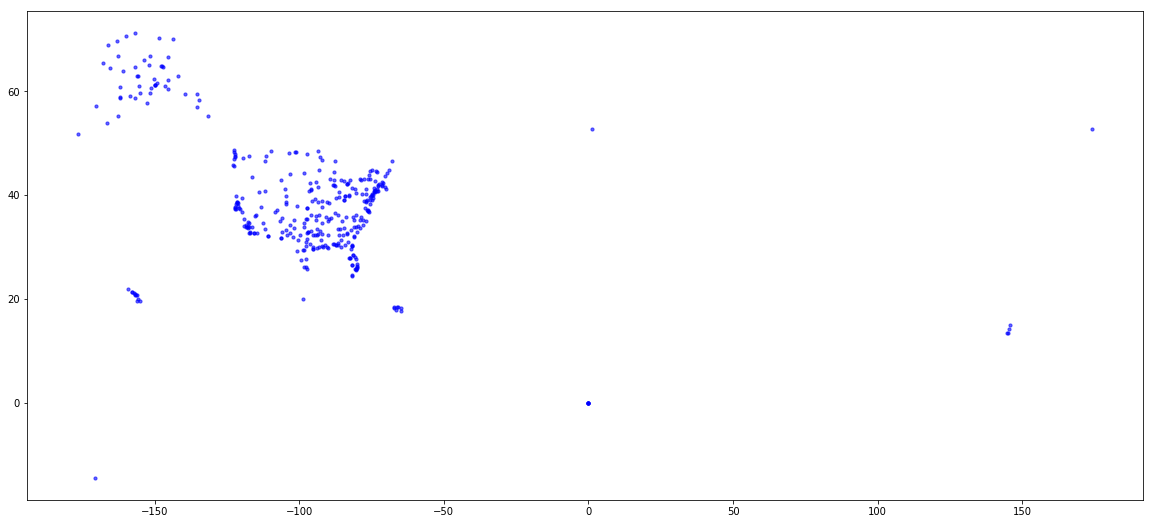In :
plt.figure(figsize=(20, 9))
x = [i for i in pos_dict.values()]
y = [i for i in pos_dict.values()]
plt.scatter(x, y)

Out:
<matplotlib.collections.PathCollection at 0x1510f0b2e8>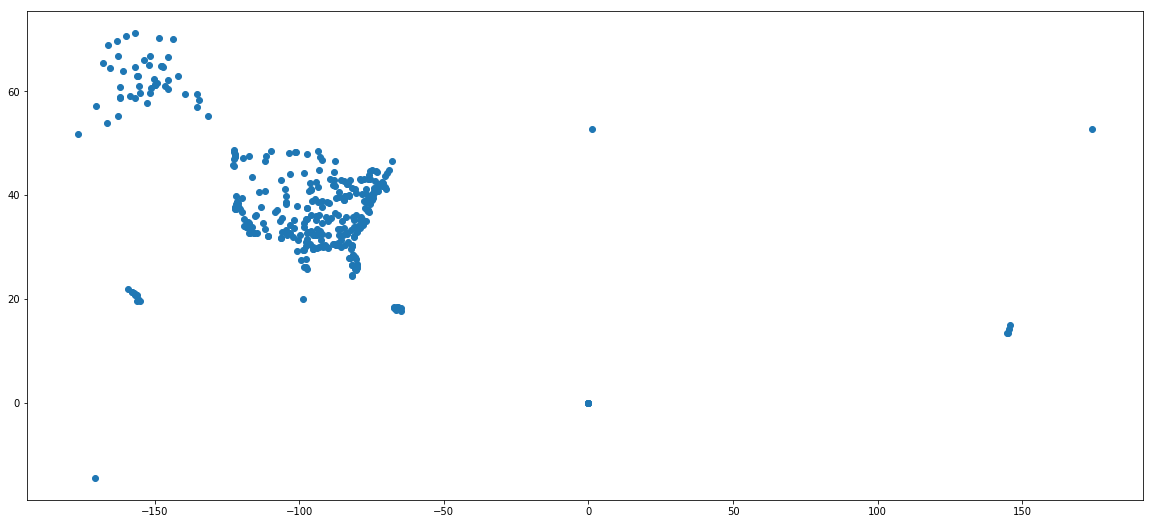### What about degree distribution of this network?¶

In :
plt.hist(list(nx.degree_centrality(pass_2015).values()))
plt.show()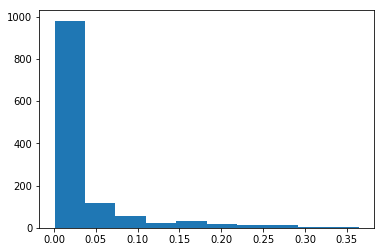Let's plot a log log plot to get a better overview of this.

In :
d = {}
for i, j in dict(nx.degree(pass_2015)).items():
if j in d:
d[j] += 1
else:
d[j] = 1
x = np.log2(list((d.keys())))
y = np.log2(list(d.values()))
plt.scatter(x, y, alpha=0.4)
plt.show()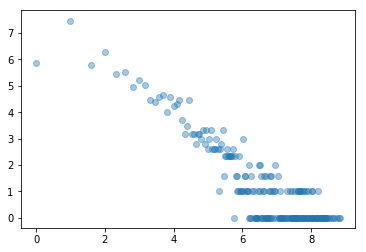### Directed Graphs¶In :
G = nx.DiGraph()

# print(G.edges())
# G
# G
# G.is_directed()
# type(G)

In :
G.add_edges_from([(1, 2), (3, 2), (4, 2), (5, 2), (6, 2), (7, 2)])
nx.draw_circular(G, with_labels=True)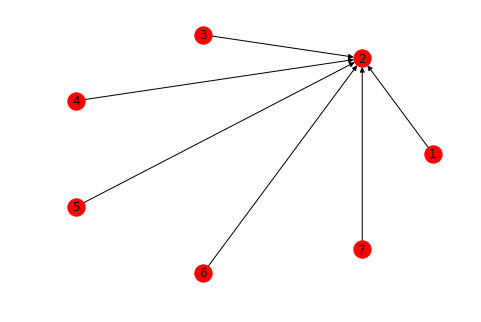In :
G.in_degree()

Out:
InDegreeView({1: 0, 2: 6, 3: 0, 4: 0, 5: 0, 6: 0, 7: 0})
In :
nx.pagerank(G)

Out:
{1: 0.0826448180198328,
2: 0.5041310918810031,
3: 0.0826448180198328,
4: 0.0826448180198328,
5: 0.0826448180198328,
6: 0.0826448180198328,
7: 0.0826448180198328}
In :
G.add_edge(5, 6)
nx.draw_circular(G, with_labels=True)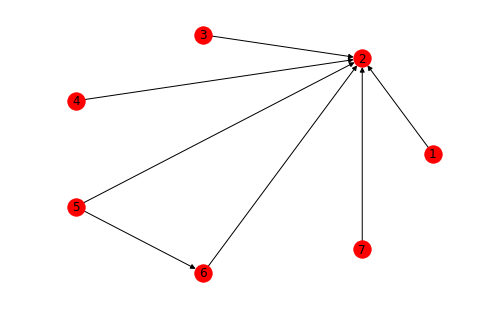In :
nx.pagerank(G)

Out:
{1: 0.08024854052495894,
2: 0.4844028780560986,
3: 0.08024854052495894,
4: 0.08024854052495894,
5: 0.08024854052495894,
6: 0.11435441931910648,
7: 0.08024854052495894}
In :
G.add_edge(2, 8)
nx.draw_circular(G, with_labels=True)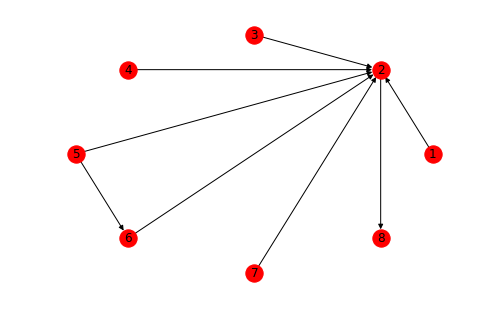In :
nx.pagerank(G)

Out:
{1: 0.05378612718073915,
2: 0.3246687852772877,
3: 0.05378612718073915,
4: 0.05378612718073915,
5: 0.05378612718073915,
6: 0.0766454192258098,
7: 0.05378612718073915,
8: 0.3297551595932067}

### Moving back to Airports¶

In :
sorted(nx.pagerank(pass_2015, weight=None).items(), key=lambda x:x, reverse=True)[:10]

Out:
[('ANC', 0.010425531156396332),
('HPN', 0.008715287139161587),
('FAI', 0.007865131822111036),
('DFW', 0.007168038232113773),
('DEN', 0.006557279519803018),
('ATL', 0.006367579588749718),
('ORD', 0.006178836107660135),
('YIP', 0.005821525504523931),
('MSP', 0.005481962582230961)]
In :
sorted(nx.betweenness_centrality(pass_2015).items(), key=lambda x:x, reverse=True)[0:10]

Out:
[('ANC', 0.28907458480586606),
('FAI', 0.08042857784594384),
('SEA', 0.06745549919241699),
('HPN', 0.06046810178534726),
('ORD', 0.045544143864829294),
('DEN', 0.038543251364241436),
('BFI', 0.03811277548952854),
('MSP', 0.03774809342340624),
('TEB', 0.036229439542316354)]
In :
sorted(nx.degree_centrality(pass_2015).items(), key=lambda x:x, reverse=True)[0:10]

Out:
[('ATL', 0.3643595863166269),
('ORD', 0.354813046937152),
('DFW', 0.3420843277645187),
('MSP', 0.3261734287987271),
('DEN', 0.31821797931583135),
('ANC', 0.3046937151949085),
('MEM', 0.29196499602227527),
('LAX', 0.2840095465393795),
('IAH', 0.28082736674622116),
('DTW', 0.27446300715990457)]

'ANC' is the airport code of Anchorage airport, a place in Alaska, and according to pagerank and betweenness centrality it is the most important airport in this network Isn't that weird? Thoughts?

Let's look at weighted version, i.e taking into account the number of people flying to these places.

In :
sorted(nx.betweenness_centrality(pass_2015, weight='weight').items(), key=lambda x:x, reverse=True)[0:10]

Out:
[('SEA', 2.2656145673621046e+50),
('ANC', 1.4996592705774285e+49),
('CDV', 1.1625885650735055e+49),
('BFI', 6.99097447478957e+48),
('PHX', 3.84552515586718e+48),
('PDX', 3.120901981185909e+48),
('LRD', 4.583490839909188e+47),
('SIT', 1.7910862390475677e+46),
('FAI', 1.137539313512378e+46)]
In :
sorted(nx.pagerank(pass_2015, weight='weight').items(), key=lambda x:x, reverse=True)[0:10]

Out:
[('ATL', 0.037535963029303135),
('ORD', 0.028329766122739346),
('SEA', 0.028274564067008245),
('ANC', 0.027127866647567035),
('DFW', 0.02570050418889442),
('DEN', 0.025260024346433315),
('LAX', 0.02394043498608451),
('PHX', 0.018373176636420224),
('CLT', 0.01780703930063076),
('LAS', 0.017649683141049966)]

## How reachable is this network?¶

We calculate the average shortest path length of this network, it gives us an idea about the number of jumps we need to make around the network to go from one airport to any other airport in this network.

In :
# nx.average_shortest_path_length(pass_2015)


Wait, What??? This network is not connected. That seems like a really stupid thing to do.

In :
list(nx.weakly_connected_components(pass_2015))

Out:
[{'WSN',
'PKB',
'A1K',
'CMI',
'GUP',
'IRK',
'HUT',
'GAL',
'05A',
'AFW',
'TNC',
'UGI',
'ALB',
'HVN',
'BOI',
'LOZ',
'RDG',
'MTM',
'EMK',
'TRI',
'TLA',
'WTL',
'PGD',
'BCT',
'SVS',
'TCL',
'JHW',
'GAI',
'B19',
'ECP',
'BBD',
'JZE',
'GNU',
'AOH',
'MVL',
'PDX',
'HAO',
'CVO',
'TVF',
'PNS',
'ANI',
'CLM',
'RKD',
'DQH',
'VIK',
'GRF',
'PDK',
'FYU',
'KOT',
'BKL',
'PWK',
'DOV',
'AZ3',
'ROC',
'GLV',
'PIR',
'KZB',
'PHF',
'A03',
'LCH',
'UXP',
'ICT',
'OAK',
'FKL',
'STP',
'OGS',
'GUS',
'NPT',
'HOB',
'YAK',
'RDU',
'AZO',
'BVY',
'TTD',
'L41',
'ISO',
'SFB',
'SLQ',
'MCO',
'OLF',
'CAK',
'HLM',
'HSH',
'GYR',
'ORV',
'RBG',
'GLH',
'KYU',
'TXK',
'JLA',
'BTM',
'HPN',
'VEL',
'LMA',
'AOS',
'TIW',
'UAM',
'NTU',
'SAN',
'EAT',
'LGU',
'TOG',
'EHM',
'NUQ',
'ME3',
'MTN',
'ZXC',
'CEF',
'YKM',
'KOY',
'MKG',
'ILG',
'WA9',
'ENN',
'CWI',
'SHH',
'MNZ',
'JLN',
'RIC',
'JAC',
'NQX',
'WBQ',
'HYL',
'KAE',
'EAR',
'BFT',
'AL7',
'LNY',
'MMI',
'EKX',
'SHG',
'EEK',
'MD1',
'N1C',
'PUW',
'AMW',
'NLG',
'LAM',
'NY7',
'FLG',
'SVA',
'SC1',
'GEK',
'LAR',
'SVH',
'NUP',
'WWT',
'BLD',
'SUU',
'FWL',
'IAB',
'JZY',
'SPS',
'EWN',
'GTY',
'KMY',
'MMT',
'DCA',
'LKP',
'A26',
'CTZ',
'KLL',
'VWK',
'LZU',
'ZXB',
'SZL',
'CEM',
'ORL',
'HRO',
'SRQ',
'OSU',
'MWH',
'XWH',
'GRB',
'SVC',
'ERI',
'OOK',
'KVC',
'ATY',
'DOF',
'CLE',
'DAY',
'TEB',
'ARB',
'MLL',
'EAU',
'APN',
'GPM',
'MXY',
'MKC',
'IWD',
'PRC',
'SDY',
'C1A',
'BHB',
'PML',
'FTG',
'KWK',
'MSS',
'PLN',
'UXK',
'LIV',
'ONH',
'HOU',
'KLG',
'A63',
'A83',
'A72',
'VZR',
'FPR',
'ARC',
'MCW',
'LAN',
'GGW',
'EFD',
'A07',
'NBG',
'UBF',
'OGD',
'CGA',
'GCC',
'MVM',
'NUL',
'KPC',
'EWR',
'ITH',
'TN5',
'AOO',
'CLP',
'GBD',
'RNT',
'DHB',
'HYA',
'LAS',
'TOL',
'OXC',
'LBF',
'WRB',
'KLS',
'KVL',
'RMP',
'KCL',
'PHL',
'AZA',
'LIT',
'AIN',
'MEM',
'LPR',
'HYG',
'JMS',
'APC',
'SFZ',
'A27',
'JZM',
'AK5',
'ISP',
'ELP',
'ESC',
'CDC',
'PA2',
'HKY',
'FAR',
'BWG',
'AEX',
'LGB',
'NTD',
'WMO',
'DQS',
'XWF',
'WRI',
'EKI',
'INT',
'DVT',
'KTN',
'FVZ',
'MCN',
'A40',
'WSJ',
'MSO',
'IKO',
'FNL',
'PTK',
'VZM',
'MOU',
'OFU',
'EWB',
'GDV',
'WA3',
'MGW',
'BLM',
'STC',
'WQU',
'SCK',
'BKC',
'SAT',
'EUG',
'PAQ',
'DNL',
'UIN',
'SQA',
'TPL',
'BFL',
'VUO',
'TOP',
'CRS',
'BDR',
'MTP',
'PKA',
'FSI',
'HDN',
'SJC',
'LBE',
'DKK',
'OME',
'VLD',
'ORI',
'LAX',
'RST',
'GCN',
'CYF',
'KXA',
'JZU',
'ZNC',
'VWD',
'LVK',
'FRY',
'ALZ',
'MKE',
'AVW',
'LAW',
'CPX',
'DDC',
'MTH',
'SUN',
'WBB',
'ZXH',
'MA5',
'PIB',
'CDW',
'ABY',
'HRL',
'GRR',
'TYE',
'SXQ',
'SBA',
'PA7',
'NHZ',
'NQA',
'TN1',
'KMO',
'AUS',
'GYY',
'OPF',
'AXN',
'KKI',
'CVG',
'LNK',
'MFD',
'SOP',
'FXE',
'TIQ',
'RFD',
'MYK',
'SWF',
'HKS',
'WVI',
'HON',
'EGV',
'ALN',
'CAE',
'MJX',
'TYS',
'SKK',
'CLT',
'PEC',
'NUI',
'SYA',
'PTU',
'GUM',
'MBS',
'TYR',
'A50',
'RBN',
'CLD',
'IAG',
'SPN',
'SGF',
'PUL',
'LBB',
'LEX',
'ENW',
'MYF',
'PGM',
'MZJ',
'BFM',
'SDF',
'SIT',
'HOP',
'NNK',
'PSE',
'OJC',
'ALW',
'CWA',
'HIB',
'LNS',
'HNM',
'PRB',
'IN1',
'PQS',
'JZI',
'1G4',
'GUF',
'ABR',
'FBK',
'CIG',
'CDR',
'BTP',
'DRT',
'DBQ',
'OSH',
'ENV',
'BIS',
'CHS',
'WST',
'SQV',
'DTR',
'XXU',
'SKW',
'IND',
'AKK',
'ZXT',
'EEN',
'VNY',
'HWD',
'HCB',
'TSP',
'HOT',
'DCU',
'FOD',
'SUX',
'VRB',
'TWF',
'MIB',
'AUW',
'FVX',
'HLN',
'PTH',
'RCA',
'LA2',
'LEW',
'MEO',
'AA8',
'FNR',
'SCE',
'GA6',
'YIP',
'ABQ',
'LOT',
'PSK',
'EXI',
'OFF',
'08A',
'LFT',
'PTV',
'FTY',
'CRW',
'HNS',
'BFD',
'GRK',
'MRI',
'A04',
'LKE',
'MRN',
'LWS',
'UMT',
'OPH',
'ORF',
'MA1',
'FWA',
'KTS',
'PSG',
'DJN',
'BWI',
'CVS',
'PQI',
'JQF',
'GBR',
'WWA',
'GKN',
'PIZ',
'LPS',
'SEA',
'IDA',
'VZA',
'SVW',
'QQY',
'MKK',
'JFK',
'BID',
'SAF',
'PVU',
'KPV',
'BMC',
'AID',
'OTZ',
'COS',
'OGG',
'CEU',
'SNP',
'TNK',
'CHD',
'NKX',
'ANB',
'DQU',
'PAE',
'MQT',
'BRO',
'PHX',
'CNY',
'PBG',
'CNO',
'ONT',
'OLV',
'AK2',
'SLC',
'LIH',
'ME1',
'NIP',
'MLB',
'FDY',
'SCC',
'MYR',
'YNG',
'GSO',
'VPS',
'SYR',
'LAF',
'SMK',
'TEK',
'BKE',
'TSG',
'AL3',
'HIK',
'ILN',
'VCV',
'LWB',
'ME2',
'OWD',
'HIE',
'YUM',
'FAT',
'WKK',
'MOD',
'SKJ',
'CHA',
'CYS',
'CHZ',
'MVW',
'OLM',
'GGG',
'ELM',
'GJT',
'FOE',
'JNU',
'SUA',
'FWH',
'BDL',
'MNN',
'FNT',
'TDZ',
'QMA',
'GED',
'MFR',
'DLG',
'BKW',
'AKP',
'RCE',
'HKB',
'UUK',
'ILI',
'FBS',
'WAA',
'CHO',
'MMU',
'RWI',
'HYS',
'ENA',
'A29',
'AKB',
'OSC',
'DEC',
'MOB',
'HPB',
'PPV',
'MDT',
'STL',
'HNL',
'OKC',
'RQV',
'SMX',
'BIF',
'KNW',
'VOK',
'LNI',
'TMB',
'MAZ',
'NJ1',
'ISM',
'RQZ',
'EDW',
'MSY',
'SBY',
'DIK',
'BFF',
'CSM',
'SJT',
'COU',
'XXN',
'SEG',
'DHN',
'GCK',
'BAF',
'VPZ',
'AZ1',
'BMI',
'AGN',
'DGB',
'DUJ',
'TLJ',
'BQK',
'KKH',
'PYM',
'AVP',
'FVQ',
'LUP',
'ABE',
'VT1',
'GSB',
'SHX',
'SSC',
'GVT',
'PA6',
'IN2',
'ATL',
'IPT',
'PA9',
'PWT',
'NYL',
'TLT',
'ASH',
'JZT',
'FOK',
'MLI',
'HUF',
'QQN',
'WYS',
'PDT',
'LAL',
'ALO',
'BYW',
'PSM',
'BIH',
'XSD',
'MTJ',
'CIK',
'ATW',
'GFL',
'FYV',
'MQJ',
'MCF',
'TUP',
'CNM',
'LKV',
'SSI',
'BUR',
'CKB',
'DE3',
'FMH',
'TDF',
'MHK',
'SLK',
'SCF',
'MKL',
'ROW',
'VWN',
'SLE',
'A35',
'MRF',
'IFP',
'MDH',
'KCG',
'BIL',
'WSB',
'PGA',
'WCR',
'JAX',
'HUS',
'CID',
'CKV',
'KOA',
'ITO',
'VZY',
'IMT',
'OGA',
'TWA',
'KCR',
'ELN',
'PPC',
'FAI',
'NGF',
'CON',
'OLU',
'GFK',
'NME',
'KWF',
'DIO',
'CDV',
'UXR',
'BHM',
'LYH',
'HOM',
'MGM',
'MTO',
'DTW',
'CRP',
'MRY',
'CLG',
'PBK',
'ZXN',
'LVD',
'EGE',
'HTO',
'MYU',
'HHH',
'PIE',
'ROA',
'SXP',
'LGA',
'RQI',
'MUE',
'JRF',
'XWA',
'VEE',
'CMX',
'CHP',
'TBN',
'APF',
'EYW',
'OCF',
'BZN',
'BTV',
'BQN',
'MEI',
'RBY',
'7AK',
'CZF',
'BPT',
'OAJ',
'MNT',
'RQN',
'KPB',
'TUL',
'ISN',
'UKI',
'MIA',
'RDB',
'EGX',
'MMH',
'TVL',
'DET',
'TIK',
'WFB',
'KSM',
'APA',
'EKO',
'MCE',
'EDE',
'JHM',
'BRD',
'COD',
'GSH',
'ONP',
'RSH',
'VZE',
'ACT',
'A43',
'WA1',
'LMT',
'DQL',
'LCK',
'CDB',
'RKS',
'TRM',
'WQZ',
'CKX',
'DMN',
'DAL',
'PBX',
'DCK',
'SOW',
'RNO',
'SHR',
'FMY',
'CXF',
'KNB',
'JRV',
'HNH',
'GSP',
'1NY',
'ALS',
'NGU',
'TN9',
'BTR',
'BLI',
'EAA',
'HGR',
'FTW',
'ROG',
'CMH',
'NPA',
'AUK',
'CIC',
'GNV',
'ACK',
'F70',
'DUA',
'DRG',
'SSW',
'MHR',
'GTR',
'TEX',
'VGC',
'LGC',
'ROP',
'MDW',
'AGS',
'GDC',
'MRC',
'HCR',
'A20',
'VQS',
'PIA',
'KWT',
'TLF',
'DTO',
'SGY',
'SVN',
'NJK',
'FRG',
'ELD',
'DMA',
'MCI',
'MI2',
'SCM',
'LSE',
'WSX',
'NKI',
'POB',
'SDM',
'RBD',
'SUS',
'FAQ',
'SIG',
'ORD',
'AWM',
'JAN',
'KY3',
'BOK',
'LRD',
'UOX',
'EDA',
'MVN',
'UNK',
'WNA',
'SBP',
'RLU',
'PCA',
'PTD',
'LCI',
'ABL',
'KGK',
'NC1',
'PBI',
'PVC',
'FCA',
'RBW',
'RIL',
'PPG',
'BRW',
'BTT',
'FLT',
'BDY',
'N1Y',
'SWO',
'MT2',
'CT1',
'TCT',
'KUK',
'SYB',
'WWP',
'A3K',
'MQI',
'SWD',
'TTN',
'IRC',
'CEC',
'BRL',
'VIS',
'PWA',
'KPR',
'PAH',
'KEH',
'CHI',
'SEE',
'HSL',
'KOZ',
'06A',
'AGC',
'VCT',
'LWM',
'KYK',
'BUF',
'ASN',
'QSO',
'BED',
'SBD',
'CGI',
'HVC',
'GAM',
'MA2',
'POU',
'CPS',
'KGX',
'ATT',
'LBL',
'BLV',
'ZXM',
'ELV',
'VDZ',
'XNA',
'ELY',
'NEW',
'TX1',
'SGR',
'IGM',
'FRD',
'SRV',
'WVL',
'SMO',
'FMN',
'BFB',
'NIN',
'ELI',
'AK6',
'AHN',
'GTF',
'CO3',
'HIO',
'WTK',
'WHD',
'OHC',
'GRI',
'SUM',
'SCH',
'STT',
'NNL',
'BMG',
'HVR',
'GEG',
'TLH',
'CIU',
'JBR',
'KBC',
'KPY',
'ZXU',
'KEK',
'ANC',
'LRU',
'RAP',
'BTI',
'MSC',
'TAL',
'BCE',
'ART',
'UST',
'PIT',
'BOS',
'NIB',
'CDL',
'IYK',
'SDX',
'DQY',
'PIP',
...},
{'SPB', 'SSB'},
{'AIK'}]

### SPB, SSB, AIK anyone?¶

In :
pass_air_data[(pass_air_data['YEAR'] == 2015) & (pass_air_data['ORIGIN'] == 'AIK')]

Out:
Unnamed: 0 YEAR ORIGIN DEST UNIQUE_CARRIER_NAME PASSENGERS
433338 433338 2015 AIK AIK {'Wright Air Service'} 0.0
In :
pass_2015.remove_nodes_from(['SPB', 'SSB', 'AIK'])

In :
nx.is_weakly_connected(pass_2015)

Out:
True
In :
nx.is_strongly_connected(pass_2015)

Out:
False

### Strongly vs weakly connected graphs.¶

In :
G = nx.DiGraph()
nx.draw(G)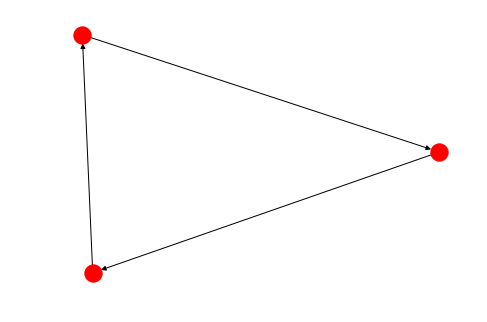In :
G.add_edge(3, 4)
nx.draw(G)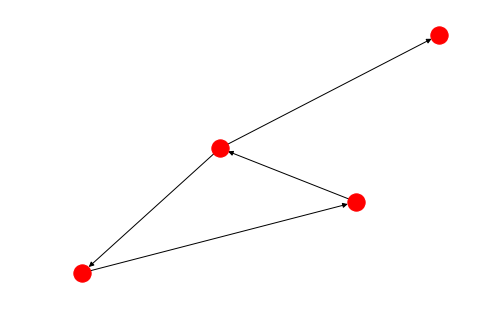In :
nx.is_strongly_connected(G)

Out:
False
In :
list(nx.strongly_connected_components(pass_2015))

Out:
[{'BCE'},
{'WA3'},
{'WA9'},
{'TIW'},
{'RQV'},
{'SUA'},
{'POU'},
{'MA5'},
{'MXF'},
{'TSP'},
{'MSV'},
{'AST'},
{'XXU'},
{'OR2'},
{'CLG'},
{'QQN'},
{'WVI'},
{'CO3'},
{'BOK'},
{'XXY'},
{'ZXC'},
{'ZXT'},
{'C1A'},
{'ELN'},
{'BKE'},
{'EKI'},
{'MA4'},
{'MI6'},
{'TMB'},
{'XWF'},
{'LZU'},
{'GUF'},
{'N1Y'},
{'DKK'},
{'TIX'},
{'MRF'},
{'WSN',
'A1K',
'PKB',
'CMI',
'GUP',
'IRK',
'HUT',
'GAL',
'TNC',
'AFW',
'05A',
'UGI',
'ALB',
'EMK',
'BOI',
'MTM',
'RDG',
'LOZ',
'HVN',
'TRI',
'WTL',
'TLA',
'SVS',
'PGD',
'BCT',
'TCL',
'JHW',
'GAI',
'BBD',
'ECP',
'JZE',
'GNU',
'AOH',
'MVL',
'PDX',
'ANI',
'TVF',
'PNS',
'DQH',
'CLM',
'CVO',
'RKD',
'VIK',
'PDK',
'FYU',
'KOT',
'BKL',
'PWK',
'DOV',
'AZ3',
'ROC',
'GLV',
'PIR',
'KZB',
'PHF',
'A03',
'HOB',
'LCH',
'ICT',
'YAK',
'XXP',
'OAK',
'OGS',
'GUS',
'FKL',
'NPT',
'TTD',
'RDU',
'AZO',
'SLQ',
'BVY',
'L41',
'ISO',
'SFB',
'MCO',
'OLF',
'CAK',
'HLM',
'HSH',
'GYR',
'ORV',
'RBG',
'KYU',
'GLH',
'TXK',
'JLA',
'BTM',
'VEL',
'LMA',
'HPN',
'AOS',
'UAM',
'NTU',
'SAN',
'EAT',
'LGU',
'TOG',
'EHM',
'ME3',
'MTN',
'CEF',
'YKM',
'KOY',
'MKG',
'ILG',
'ENN',
'CWI',
'SHH',
'WBQ',
'MNZ',
'JLN',
'NQX',
'RIC',
'JAC',
'KAE',
'HYL',
'EAR',
'AL7',
'BFT',
'LNY',
'EEK',
'SHG',
'MMI',
'EKX',
'AMW',
'N1C',
'MD1',
'PUW',
'NLG',
'LAM',
'NY7',
'FLG',
'SVA',
'SC1',
'GEK',
'LAR',
'NUP',
'SVH',
'WWT',
'FWL',
'SUU',
'BLD',
'IAB',
'JZY',
'SPS',
'EWN',
'GTY',
'KMY',
'DCA',
'LKP',
'CTZ',
'KLL',
'ZXB',
'VWK',
'SZL',
'CEM',
'HRO',
'XWH',
'OSU',
'ORL',
'MWH',
'SRQ',
'GRB',
'DOF',
'SVC',
'ERI',
'KVC',
'ATY',
'OOK',
'CLE',
'DAY',
'ARB',
'TEB',
'MLL',
'EAU',
'GPM',
'APN',
'MXY',
'SDY',
'IWD',
'MKC',
'PRC',
'BHB',
'PML',
'FTG',
'KWK',
'MSS',
'PLN',
'UXK',
'LIV',
'ONH',
'HOU',
'KLG',
'A63',
'A83',
'A72',
'VZR',
'ARC',
'MCW',
'LAN',
'GGW',
'EFD',
'A07',
'CGA',
'NUL',
'UBF',
'OGD',
'GCC',
'MVM',
'KPC',
'EWR',
'TN5',
'ITH',
'AOO',
'RNT',
'GBD',
'DHB',
'CLP',
'HYA',
'LAS',
'TOL',
'LBF',
'WRB',
'OXC',
'KVL',
'KLS',
'RMP',
'KCL',
'PHL',
'AZA',
'LIT',
'AIN',
'MEM',
'AK5',
'JZM',
'A27',
'HYG',
'SFZ',
'JMS',
'APC',
'LPR',
'ISP',
'ELP',
'CDC',
'ESC',
'PA2',
'HKY',
'FAR',
'BWG',
'AEX',
'NTD',
'LGB',
'WMO',
'DQS',
'DVT',
'WRI',
'A40',
'INT',
'FVZ',
'KTN',
'MCN',
'WSJ',
'MSO',
'OFU',
'PTK',
'FNL',
'MOU',
'VZM',
'EWB',
'GDV',
'WQU',
'BLM',
'STC',
'MGW',
'SCK',
'BKC',
'SAT',
'EUG',
'PAQ',
'DNL',
'UIN',
'SQA',
'TPL',
'BFL',
'VUO',
'TOP',
'CRS',
'BDR',
'MTP',
'PKA',
'FSI',
'HDN',
'SJC',
'LBE',
'OME',
'VLD',
'ORI',
'CYF',
'LAX',
'RST',
'GCN',
'JZU',
'ZNC',
'VWD',
'LVK',
'FRY',
'ALZ',
'MKE',
'AVW',
'LAW',
'CPX',
'ZXH',
'MTH',
'SUN',
'DDC',
'WBB',
'PIB',
'ABY',
'HRL',
'GRR',
'TYE',
'SXQ',
'PA7',
'SBA',
'NHZ',
'NQA',
'TN1',
'KMO',
'AUS',
'AXN',
'GYY',
'OPF',
'KKI',
'CVG',
'LNK',
'SOP',
'MFD',
'FXE',
'TIQ',
'RFD',
'MYK',
'SWF',
'HKS',
'HON',
'EGV',
'ALN',
'CAE',
'MJX',
'TYS',
'SKK',
'CLT',
'PEC',
'NUI',
'SYA',
'PTU',
'GUM',
'MBS',
'TYR',
'A50',
'RBN',
'CLD',
'IAG',
'PUL',
'SGF',
'SPN',
'LBB',
'LEX',
'ENW',
'MYF',
'PGM',
'BFM',
'MZJ',
'SDF',
'SIT',
'HOP',
'NNK',
'ALW',
'OJC',
'PSE',
'CWA',
'HIB',
'LNS',
'HNM',
'PRB',
'IN1',
'PQS',
'JZI',
'1G4',
'ABR',
'FBK',
'CIG',
'CDR',
'BTP',
'DBQ',
'SQV',
'OSH',
'ENV',
'BIS',
'CHS',
'WST',
'RBW',
'DTR',
'DRT',
'SKW',
'IND',
'AKK',
'EEN',
'VNY',
'HCB',
'HWD',
'HOT',
'DCU',
'FOD',
'SUX',
'VRB',
'TWF',
'MIB',
'AUW',
'FVX',
'HLN',
'PTH',
'RCA',
'AA8',
'LA2',
'MEO',
'LEW',
'FNR',
'SCE',
'GA6',
'LOT',
'ABQ',
'YIP',
'PSK',
'EXI',
'08A',
'LFT',
'PTV',
'FTY',
'CRW',
'A04',
'HNS',
'BFD',
'GRK',
'LKE',
'MRI',
'MRN',
'OPH',
'LWS',
'UMT',
'ORF',
'FWA',
'KTS',
'PSG',
'BWI',
'CVS',
'GBR',
'JQF',
'PQI',
'WWA',
'GKN',
'PIZ',
'LPS',
'SEA',
'IDA',
'VZA',
'SVW',
'QQY',
'MKK',
'JFK',
'BID',
'SAF',
'PVU',
'KPV',
'BMC',
'AID',
'OTZ',
'SNP',
'COS',
'CEU',
'OGG',
'TNK',
'CHD',
'NKX',
'ANB',
'DQU',
'PAE',
'MQT',
'AK2',
'PHX',
'CNY',
'BRO',
'OLV',
'ONT',
'PBG',
'CNO',
'SLC',
'LIH',
'ME1',
'SCC',
'FDY',
'MLB',
'NIP',
'MYR',
'YNG',
'GSO',
'VPS',
'SYR',
'LAF',
'SMK',
'TEK',
'TSG',
'AL3',
'HIK',
'ILN',
'VCV',
'ME2',
'OWD',
'LWB',
'YUM',
'FAT',
'SKJ',
'MOD',
'WKK',
'CHA',
'CYS',
'MVW',
'CHZ',
'OLM',
'GGG',
'ELM',
'JNU',
'GJT',
'FOE',
'FWH',
'BDL',
'MNN',
'FNT',
'TDZ',
'AUK',
'GED',
'DLG',
'QMA',
'AKP',
'HKB',
'UUK',
'BKW',
'ILI',
'MFR',
'RCE',
'WAA',
'CHO',
'HYS',
'ENA',
'MMU',
'RWI',
'A29',
'AKB',
'OSC',
'DEC',
'HPB',
'MOB',
'PPV',
'HNL',
'STL',
'MDT',
'OKC',
'SMX',
'BIF',
'KNW',
'VOK',
'LNI',
'MAZ',
'NJ1',
'RQZ',
'EDW',
'MSY',
'SBY',
'DIK',
'A26',
'BFF',
'XXN',
'COU',
'SJT',
'CSM',
'SEG',
'DHN',
'GCK',
'BAF',
'AZ1',
'VPZ',
'AGN',
'DGB',
'BMI',
'DUJ',
'TLJ',
'KKH',
'BQK',
'PYM',
'AVP',
'FVQ',
'LUP',
'ABE',
'VT1',
'SHX',
'SSC',
'IN2',
'GVT',
'PA6',
'ATL',
'PWT',
'PA9',
'IPT',
'TLT',
'NYL',
'ASH',
'JZT',
'FOK',
'WYS',
'MLI',
'HUF',
'PDT',
'LAL',
'ALO',
'BYW',
'BIH',
'XSD',
'CIK',
'PSM',
'MTJ',
'ATW',
'GFL',
'FYV',
'MQJ',
'MCF',
'CNM',
'LKV',
'TUP',
'SSI',
'BUR',
'DE3',
'TDF',
'FMH',
'CKB',
'MHK',
'SLK',
'SCF',
'MKL',
'ROW',
'A35',
'VWN',
'SLE',
'IFP',
'MDH',
'KCG',
'BIL',
'WSB',
'HUS',
'PGA',
'JAX',
'WCR',
'CID',
'VZY',
'CKV',
'KOA',
'ITO',
'IMT',
'OGA',
'TWA',
'KCR',
'PPC',
'FAI',
'CON',
'OLU',
'GFK',
'NME',
'KWF',
'DIO',
'CDV',
'UXR',
'BHM',
'LYH',
'HOM',
'PBK',
'MGM',
'DTW',
'ZXN',
'CRP',
'MRY',
'MTO',
'LVD',
'EGE',
'HTO',
'MYU',
'HHH',
'PIE',
'ROA',
'SXP',
'LGA',
'RQI',
'MUE',
'JRF',
'XWA',
'VEE',
'CMX',
'CHP',
'TBN',
'APF',
'EYW',
'OCF',
'RBY',
'7AK',
'BTV',
'KPB',
'BZN',
'BQN',
'MEI',
'BPT',
'OAJ',
'RQN',
'CZF',
'MNT',
'TUL',
'UKI',
'ISN',
'MIA',
'RDB',
'EGX',
'MMH',
'DET',
'WFB',
'KSM',
'TVL',
'TIK',
'EKO',
'APA',
'MCE',
'EDE',
'JHM',
'BRD',
'ONP',
'GSH',
'COD',
'ROP',
'RSH',
'A43',
'ACT',
'VZE',
'WA1',
'LMT',
'DQL',
'LCK',
'CDB',
'RKS',
'TRM',
'WQZ',
'CKX',
'DMN',
'DAL',
'PBX',
'DCK',
'SOW',
'SHR',
'CXF',
'FMY',
'RNO',
'KNB',
'JRV',
'EAA',
'HNH',
'KXA',
'GSP',
'TN9',
'1NY',
'BTR',
'ALS',
'BLI',
'HGR',
'FTW',
'NGU',
'CMH',
'ROG',
'CIC',
'GNV',
'ACK',
'DUA',
'DRG',
'SSW',
'MHR',
'GTR',
'VGC',
'TEX',
'A20',
'KWT',
'AGS',
'MRC',
'MDW',
'HCR',
'LGC',
'GDC',
'VQS',
'PIA',
'TLF',
'DTO',
'SGY',
'FAQ',
'ELD',
'DMA',
'SVN',
'FRG',
'MCI',
'MI2',
'SCM',
'LSE',
'WSX',
'NKI',
'POB',
'RBD',
'SDM',
'SUS',
'SIG',
'ORD',
'JAN',
'LRD',
'UOX',
'EDA',
'MVN',
'UNK',
'WNA',
'RLU',
'PCA',
'PTD',
'SBP',
'LCI',
'ABL',
'KGK',
'NC1',
'PBI',
'PVC',
'BTT',
'BRW',
'RIL',
'IKO',
'FLT',
'KUK',
'FCA',
'MT2',
'SYB',
'PPG',
'BDY',
'CT1',
'SWO',
'A3K',
'TCT',
'WWP',
'MQI',
'SWD',
'TTN',
'IRC',
'BRL',
'CEC',
'PWA',
'KPR',
'VIS',
'KEH',
'PAH',
'CHI',
'HSL',
'SEE',
'KOZ',
'06A',
'VCT',
'AGC',
'LWM',
'KYK',
'BUF',
'ASN',
'QSO',
'BED',
'SBD',
'CGI',
'HVC',
'GAM',
'MA2',
'ATT',
'CPS',
'KGX',
'LBL',
'BLV',
'ZXM',
'VDZ',
'ELV',
'XNA',
'ELY',
'TX1',
'NEW',
'SGR',
'FRD',
'IGM',
'SRV',
'WVL',
'BFB',
'FMN',
'SMO',
'NIN',
'WHD',
'AK6',
'AHN',
'GTF',
'HIO',
'ELI',
'WTK',
'OHC',
'GRI',
'SCH',
'STT',
'NNL',
'BMG',
'HVR',
'GEG',
'TLH',
'CIU',
'JBR',
'KPY',
'KBC',
'ZXU',
'KEK',
'ANC',
'LRU',
'RAP',
'NIB',
'CDL',
'TAL',
'MSC',
'ART',
'UST',
'BOS',
'PIT',
'FBS',
'BTI',
'IYK',
'SDX',
'PIP',
'IAN',
'GVL',
'HTS',
'GGE',
'SHV',
'GUC',
'PVD',
'RSJ',
'MCG',
'CKD',
'FLO',
'KTB',
'BTL',
'BJI',
'TKJ',
'BNA',
'DE2',
'RSN',
'CBE',
'LFI',
'FSM',
'SDP',
'TVC',
'OWB',
'KLW',
'AIA',
'WA6',
'PUB',
'PCT',
'MFE',
'STP',
'PHO',
'AMA',
'TKA',
'DLH',
'KKA',
'DSM',
'OLE',
'BFI',
'GBH',
'FSD',
'RDD',
'BJC',
'PNE',
'6B0',
'MQY',
'ABI',
'WRG',
'MHT',
'ISS',
'MIE',
...},
{'LSV'},
{'FFO'},
{'NGF'},
{'NPA'},
{'NUQ'},
{'UXP'},
{'GSB'},
{'VWX'},
{'PAM'},
{'GRF'},
{'FPR'},
{'HAO'},
{'NJK'},
{'DJN'},
{'SUM'},
{'NBG'},
{'OFF'},
{'AWM'},
{'ISM'},
{'KY3'},
{'MMT'},
{'QMN'},
{'CDW'},
{'MA1'},
{'HIE'},
{'B19'},
{'F70'},
{'DQY'}]
In :
pass_air_data[(pass_air_data['YEAR'] == 2015) & (pass_air_data['DEST'] == 'TSP')]

Out:
Unnamed: 0 YEAR ORIGIN DEST UNIQUE_CARRIER_NAME PASSENGERS
450245 450245 2015 ONT TSP {'Federal Express Corporation'} 0.0
In :
pass_2015_strong = max(nx.strongly_connected_component_subgraphs(pass_2015), key=len)

In :
len(pass_2015_strong)

Out:
1190
In :
nx.average_shortest_path_length(pass_2015_strong)

Out:
3.174661992635574

#### Exercise! (Actually this is a game :D)¶

How can we decrease the avg shortest path length of this network?

Think of an effective way to add new edges to decrease the avg shortest path length. Let's see if we can come up with a nice way to do this, and the one who gets the highest decrease wins!!!

The rules are simple:

• You can't add more than 2% of the current edges( ~500 edges)

[10 mins]

In :
sort_degree = sorted(nx.degree_centrality(pass_2015_strong).items(), key=lambda x:x, reverse=True)
top_count = 0
for n, v in sort_degree:
count = 0
for node, val in sort_degree:
if node != n:
count += 1
if count == 25:
break
top_count += 1
if top_count == 20:
break

In :
nx.average_shortest_path_length(pass_2015_strong)

Out:
3.0887646564092415

### What about airlines? Can we find airline specific reachability?¶

In :
passenger_graph['JFK']['SFO']

Out:
{'PASSENGERS': 1179941.0,
'UNIQUE_CARRIER_NAME': "{'Delta Air Lines Inc.', 'Virgin America', 'American Airlines Inc.', 'Sun Country Airlines d/b/a MN Airlines', 'JetBlue Airways', 'Vision Airlines', 'United Air Lines Inc.'}",
'YEAR': 2015}
In :
def str_to_list(a):
return a[1:-1].split(', ')

In :
for i in str_to_list(passenger_graph['JFK']['SFO']['UNIQUE_CARRIER_NAME']):
print(i)

'Delta Air Lines Inc.'
'Virgin America'
'American Airlines Inc.'
'Sun Country Airlines d/b/a MN Airlines'
'JetBlue Airways'
'Vision Airlines'
'United Air Lines Inc.'

In :
%%time
for origin, dest in passenger_graph.edges():
for key in passenger_graph[origin][dest]:
passenger_graph[origin][dest][key]['airlines'] = str_to_list(passenger_graph[origin][dest][key]['UNIQUE_CARRIER_NAME'])

CPU times: user 41.4 s, sys: 223 ms, total: 41.6 s
Wall time: 41.8 s


### Exercise¶

Play around with United Airlines network.

• Extract a network for United Airlines flights from the metagraph passenger_graph for the year 2015
• Make sure it's a weighted network, where weight is the number of passengers.
• Find the number of airports and connections in this network
• Find the most important airport, according to PageRank and degree centrality.
In :
united_network = nx.DiGraph()
for origin, dest in passenger_graph.edges():
if 25 in passenger_graph[origin][dest]:
if "'United Air Lines Inc.'" in passenger_graph[origin][dest]['airlines']:

In :
len(united_network)

Out:
134
In :
len(united_network.edges())

Out:
1097
In :
sorted(nx.pagerank(united_network, weight='weight').items(), key=lambda x:x, reverse=True)[0:10]

Out:
[('ORD', 0.09229686412847604),
('DEN', 0.06951536832747153),
('LAX', 0.05547087049076451),
('SFO', 0.04520212084776551),
('IAH', 0.04398280474534501),
('EWR', 0.03754166218607773),
('ATL', 0.034778751100523315),
('DFW', 0.03419728511206331),
('PHX', 0.025365344038763896),
('MSP', 0.022269133819322266)]
In :
sorted(nx.degree_centrality(united_network).items(), key=lambda x:x, reverse=True)[0:10]

Out:
[('ORD', 1.1729323308270676),
('DEN', 0.9172932330827067),
('IAH', 0.8195488721804511),
('EWR', 0.744360902255639),
('SFO', 0.5789473684210527),
('LAX', 0.5338345864661653),
('CLE', 0.39849624060150374),
('STL', 0.3609022556390977),
('PHL', 0.3308270676691729)]

### Exercise¶

We are in Cleveland so what should we do?

Obviously we will make a time series of number of passengers flying out of Cleveland with United Airlines over the years.

There are 2 ways of doing it.

• Create a new multidigraph specifically for this exercise.

OR

• exploit the pass_air_data dataframe.
In :
pass_air_data[(pass_air_data.ORIGIN == 'CLE') &
(pass_air_data.UNIQUE_CARRIER_NAME.str.contains('United Air Lines Inc.'))
].groupby('YEAR')['PASSENGERS'].sum().plot()

Out:
<matplotlib.axes._subplots.AxesSubplot at 0x1532b4dd68>